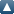# Browse by Document typeUp a level
 Export as ASCII CitationBibTeXDublin CoreEP3 XMLEndNoteHTML CitationJSONMETSObject IDsOpenURL ContextObjectRDF+N-TriplesRDF+N3RDF+XMLReferReference Manager
Group by: Date | Authors | No Grouping
Number of items: 34.

## March 2010

Balasubramanian, Krishnan (2010) Computational & mathematical concepts in arts and science. Learning Curve (14). pp. 23-25.

Behar, S C (2010) The hypocrisy in math. Learning Curve (14). pp. 109-110.

Chudgar, Amita (2010) Learning to add: are we subtracting the importance of the home environment? Learning Curve (14). pp. 114-116.

Dewan, Hridaykant (2010) Pedagogy of mathematics. Learning Curve (14). pp. 16-22.

Gananath, S N (2010) Digital resources for mathematics teachers. Learning Curve (14). pp. 133-135.

Gangola, Anant (2010) Unsung heroes: what is it that makes them stand out? Learning Curve (14). pp. 124-125.

Gupta, Arvind (2010) Broomstick tables. Learning Curve (14). pp. 81-82.

Gupta, Arvind (2010) Maths missionary – P. K. Srinivasan. Learning Curve (14). pp. 111-113.

Jagadeeshan, Shashidhar (2010) A culture of enjoying mathematics. Learning Curve (14). pp. 7-11.

Karopady, D. D. (2010) What ails mathematics teaching? Learning Curve (14). pp. 42-47.

Kingdon, Geeta Gandhi and Banerji, Rukmini (2010) How sound are our mathematics teachers? Learning Curve (14). pp. 52-55.

Kumar, Uma Hari (2010) The value of drill and practice in math: a perspective. Learning Curve (14). pp. 69-71.

Kumar, V S (2010) Engineers, accountants And mathematics. Learning Curve (14). pp. 126-128.

Mukherjee, Amitabha (2010) The nature of mathematics and its relation 2 to school education. Learning Curve (14). pp. 12-15.

Mukunda, Kamala (2010) Important concepts in primary school mathematics. Learning Curve (14). pp. 37-39.

Nadig, Devika and Gupta, Vijay (2010) Meaningful teaching of mathematics. Learning Curve (14). pp. 57-59.

Naik, Arun (2010) Concept attainment model. Learning Curve (14). pp. 60-62.

Narayan, Devika (2010) Math for the non-mathematical soul. Learning Curve (14). pp. 122-123.

Prasad, Indu (2010) Confessions of a victim of mathematics. Learning Curve (14). pp. 117-119.

Prasad, Indu (2010) Mathematics and the national curriculum framework. Learning Curve (14). pp. 34-36.

Raghavan, Neeraja (2010) Two books worth a read. Learning Curve (14). pp. 130-132.

Rajagopalan, Sridhar (2010) Insights about student learning from an adaptive learning math program. Learning Curve (14). pp. 93-99.

Ram, Shwetha (2010) The elusive “Mr.Math”. Learning Curve (14). pp. 120-121.

Ramachandran, Nat (2010) Mother's math and the art of estimation. Learning Curve (14). pp. 40-41.

S. Rathore, Abhishek and Sarangi, Falguni (2010) Response analysis: understanding children from their frame of reference. Learning Curve (14). pp. 88-92.

Sarukkai, Sundar (2010) Ancient India and mathematics. Learning Curve (14). pp. 48-51.

Satry, V S S (2010) Fold paper and learn mathematics. Learning Curve (14). pp. 77-80.

Sharma, Ekta (2010) Dialogue in math teaching: realities and challenges. Learning Curve (14). pp. 63-65.

Shenoy, Sulata (2010) 2 + 2 = ? a case for early identification of dyscalculia. Learning Curve (14). pp. 72-76.

Shirali, Shailesh (2010) Number: the role of pattern and play in its teaching. Learning Curve (14). pp. 30-33.

Subramaniam, k (2010) Culture in the learning of mathematics. Learning Curve (14). pp. 26-28.

Subramanian, H (2010) Ten great mathematicians. Learning Curve (14). pp. 101-108.

Suresh, Meena (2010) The role of concrete experiences in learning primary school math. Learning Curve (14). pp. 66-68.

Turner, Ross (2010) Lessons from the international PISA project. Learning Curve (14). pp. 84-87.

This list was generated on Sat Jun 3 05:52:59 2023 UTC.